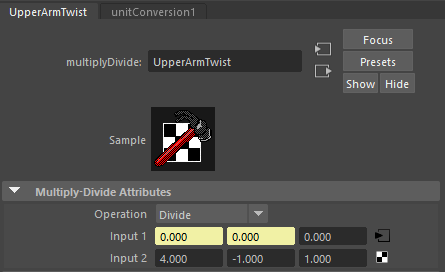Search
•Alexander Wagner

# Distributed Twist Joints

Depending on the process you use for creating a character rig. You may have noticed that the arms and legs do not deform as realistically as you would like. These rigs could benefit from a concept called distributed twist joints. The idea is that this rigging technique emulates the rate of twisting involved in the hand relative to the elbow.

As a fun example, you can lay your forearm flat on table, palm down. Now twist your hand, palm facing up and notice how much your elbow is twisting relative to your wrist. In reality, your elbow doesn't twist at all! This is the movement that will be emulated in a distributed twist joint setup.

Let's Begin!

## Creating the joints for the twist dispersion rig

Usually when we will implement this kind of rig we will have both an IK and FK that drives the twist dispersion. For the sake of simplicity we will only setup an FK arm rig to drive the twist joints in this demonstration.Simple FK arm setup with animation controls

The next step will be to create our twist joints. What we want to do is query the translate x value of the elbow joint. Then divide that length by the number of twist joints we want in the upper arm section. This will be our length average. Using that averaged length, create the upper twist joints on top of the FK joints. Repeat this process for the lower arm. Add the new wrist end joint in the same position as FK wrist end joint. In my experience I have found that creating an extra joint in the same position as the shoulder is needed to help the negative twist. (explained later in tutorial) This will be the offset twist joint the other twist joints will be parented under.

We should have a twist joint setup like so.Twist joints shown in fuchsia.

## Setting up the connections to drive the upper arm twist dispersion

Now the fun part! We get to work in the node editor. For setting up the upper arm we need to select the FK shoulder joint, and the upper arm twist joints. Populate the node editor with the selected joints. Create a Multiply/Divide node and set the operation to Divide. Take the FK shoulder joint's Rotate X channel and connect it to the Multiply/Divide nodes Input 1X and Input 1Y.Example Values for channels in the Multiply/Divide node.

Next we need to set the Input 2X and 2Y channels. The Input 2X needs to be set to the amount of twist joints in the upper arm. The Input 2Y needs to be set to -1. This counters the rotation of the driving joint and makes the twist nullified for the first twist joint. The values down the chain should look like this. Starting at the shoulder: zero twist, then 0.25, 0.50, 0.75.

Now we need to connect the output of the Multiply/Divide node to the twist joints. Take the Output Y channel and connect it to the first twist joint's input Rotate X channel. This will be the negative rotation values. Then connect the Output X into the remaining twist joints for the upper arm. Note: A conversion node will be created. Instead of creating a conversion node for each joint. Just use the first conversion node and use that output for the twist joints.Node setup for finished upper arm twist dispersion.

## Setting up the connections to drive the lower arm twist dispersion

Lets finish up with the lower arm. select the wrist and lower arm twist joints and populate the node editor with them. This setup will be a little different than the upper arm setup in that I found it doesn't need a negative value for the first twist joint. We will need to create another Multiply/Divide node. Set its Input 2X to the number of twist joints in the lower arm. Connect the output Rotate X of FK wrist joint to the Input 1X channel of the Multiply/Divide node. Now connect the Output X channel of the Multiply/Divide node to the lower twist joints, except the first twist joint.Node setup for finished lower arm twist dispersion.

To finish off the rig we need to constrain the twist joints to the appropriate FK joints. Parent Constrain the offset upper twist joint to the FK shoulder joint. Constrain the first lower twist joint to FK elbow joint. Finally constrain the twist wrist joint to the FK wrist joint.

The final results of the distributed twist arm rig should look like this! This is just a very basic setup. A more advanced rig will involve matrix math for handling input and output values. vector math to determine negative or positive rotation, and quaternion math for twist angle extraction. An important function to look forward to in advanced twist dispersion is getting rid of the dreaded flipping when ever we pass 180 degrees of rotation.

And of course this whole process can be automated with scripting. We can even write a custom twist dispersion node with Maya Python API, and even better C++ for maximum efficiency!